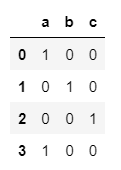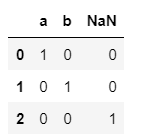top of page

# pandas techniques

## 1) Apply function

### Objects passed to the function are Series objects whose index is either the DataFrame’s index (axis=0) or the DataFrame’s columns (axis=1). By default (result_type=None)

##### first we add the needed libraries , this is the first step when using pandas functions
```
import pandas as pd
import numpy as np```

##### creating a dataframe
```df = pd.DataFrame([[1,2,3]]*5 , columns = ['A','B','C'])
print(df)```
###### output:
```   A  B  C
0  1  2  3
1  1  2  3
2  1  2  3
3  1  2  3
4  1  2  3```

##### applying apply function per column
`df.apply(np.sum , axis =0)`
###### output:
```A     5
B    10
C    15
dtype: int64```

##### applying apply function per row
`df.apply(np.sum , axis =1)`
###### output:
```0    6
1    6
2    6
3    6
4    6
dtype: int64```

## 2) Boolean indexing

### Boolean Indexing is used if user wants to filter the values of a column based on conditions from another set of columns. For instance, we want a list of all students who are not scholars and got a loan. Boolean indexing can support here.

##### in python 0 is false , 1 is true and vice versa
`0==False`
###### output:
`True`

```c = 10
(c > 1) + (c<20) +(c == 12)```
`2`

##### A boolean test can be used as an index for an index for an array or tuple
```state = True
state = (True , False)[state]
state```
`False`

## 3) Is null function

### This function takes a scalar or array-like object and indicates whether values are missing .

```import pandas as pd
import numpy as np```
`pd.isna('dog')`
`False`
##### Is pd.na a null value?
`pd.isna(pd.NA)`
`True`
##### Is np.nan a null value?
`pd.isna(np.nan)`
`True`
##### construct an array with null values , and pass it to isna function
```array = np.array([[1, np.nan, 3], [4, 5, np.nan]])
pd.isna(array)

```
###### output:
```array([[False,  True, False],
[False, False,  True]])```

##### construct a dataframe , and pass it to isna function
```df = pd.DataFrame([['ant', 'bee', 'cat'], ['dog', None, 'fly']])
pd.isna(df)```
###### output:`pd.isna(df)`
###### output:
```0    False
1     True
Name: 1, dtype: bool```

## 4) Get dummies

### Convert categorical variable into dummy/indicator variables.

##### convert the list into dummy variables
```import pandas as pd
import numpy as np
s = pd.Series(list('abca'))
pd.get_dummies(s)```
###### output:##### convert the list into dummy variables
```s1 = ['a', 'b', np.nan]
pd.get_dummies(s1)```
###### output:##### it includes the null values when converting to dummy varaibles
`pd.get_dummies(s1, dummy_na=True)`
###### output:## 5) Cut function

### The input array to be binned. Must be 1-dimensional.

##### Discretize into three equal-sized bins.
```import pandas as pd
import numpy as np
pd.cut(np.array([1, 7, 5, 4, 6, 3]), 3)```
###### output:
```[(0.994, 3.0], (5.0, 7.0], (3.0, 5.0], (3.0, 5.0], (5.0, 7.0], (0.994, 3.0]]
Categories (3, interval[float64, right]): [(0.994, 3.0] < (3.0, 5.0] < (5.0, 7.0]]```
##### Discretize into three equal-sized bins ,
`pd.cut(np.array([1, 7, 5, 4, 6, 3]), 3, retbins=True)`
###### output:
```([(0.994, 3.0], (5.0, 7.0], (3.0, 5.0], (3.0, 5.0], (5.0, 7.0], (0.994, 3.0]]
Categories (3, interval[float64, right]): [(0.994, 3.0] < (3.0, 5.0] < (5.0, 7.0]],
array([0.994, 3.   , 5.   , 7.   ]))```

```pd.cut(np.array([1, 7, 5, 4, 6, 3]),

```
###### output:
```['bad', 'good', 'medium', 'medium', 'good', 'bad']
Categories (3, object): ['bad' < 'medium' < 'good']```